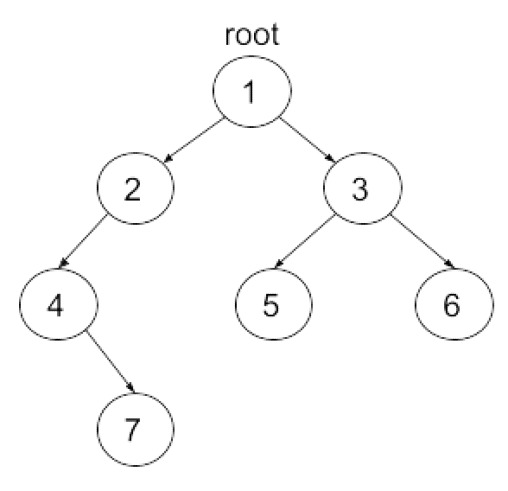New update is available. Click here to update.

# Maximum Level Sum

Last Updated: 10 Nov, 2020
Difficulty: Easy

## PROBLEM STATEMENT

#### Given a Binary Tree with integer nodes, your task is to find the maximum level sum among all the levels in the Binary Tree. The sum of any level in a tree is the sum of all the nodes present at that level.

##### Input Format:
``````The first line will contain 'T', the number of test cases.

The only line of each test case contains elements in the level order form. The line consists of values of nodes separated by a single space. In case a node is null, we take -1 on its place.

For example, the input for the tree depicted in the below image would be :
````````````1
2 3
4 -1 5 6
-1 7 -1 -1 -1 -1
-1 -1
``````

#### Explanation :

``````Level 1 :
The root node of the tree is 1

Level 2 :
Left child of 1 = 2
Right child of 1 = 3

Level 3 :
Left child of 2 = 4
Right child of 2 = null (-1)
Left child of 3 = 5
Right child of 3 = 6

Level 4 :
Left child of 4 = null (-1)
Right child of 4 = 7
Left child of 5 = null (-1)
Right child of 5 = null (-1)
Left child of 6 = null (-1)
Right child of 6 = null (-1)

Level 5 :
Left child of 7 = null (-1)
Right child of 7 = null (-1)

The first not-null node(of the previous level) is treated as the parent of the first two nodes of the current level. The second not-null node (of the previous level) is treated as the parent node for the next two nodes of the current level and so on.

The input ends when all nodes at the last level are null(-1).
``````
##### Note :
``````The above format was just to provide clarity on how the input is formed for a given tree.
The sequence will be put together in a single line separated by a single space. Hence, for the     above-depicted tree, the input will be given as:

1 2 3 4 -1 5 6 -1 7 -1 -1 -1 -1 -1 -1
``````
##### Output Format:
``````For each test case, return a single integer denoting the maximum level sum.

The output for each test case is printed in a separate line.
``````
##### Note:
``````You do not need to print anything, it has already been taken care of. Just implement the given function.
``````
##### Constraints:
``````1 <= T <= 100
1 <= N <= 1000
-10^5  <= DATA <= 10^5 and DATA != -1

Where ‘N’ is the number of nodes, 'DATA' is the value of nodes in the given tree.

Time limit: 1 sec
``````## Approach 1

Let’s traverse the given Binary tree using Recursion.The idea is to recursively store the sum of nodes of every level separately in a map.

• Take a map to store the sum of each level.
• The recursive function has ‘ROOT’, current level, and map as its parameters.
• Base Condition:  If ‘ROOT’ is NULL, return.
• Add the value of the current node to the value mapped to the current level in the map.
• Recur for the left and the right child by passing the value of ‘LEVEL’ as ‘LEVEL’ + 1 i.e. present ‘LEVEL’ + 1 (for next level).
• Now traverse the map to find the maximum value among all ‘LEVEL’ sums.
• Print the maximum value found in step 2.